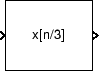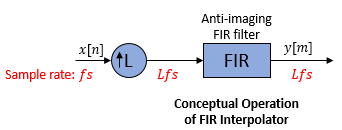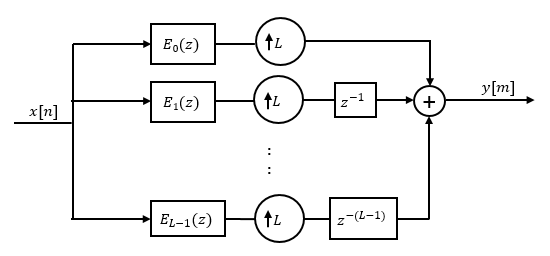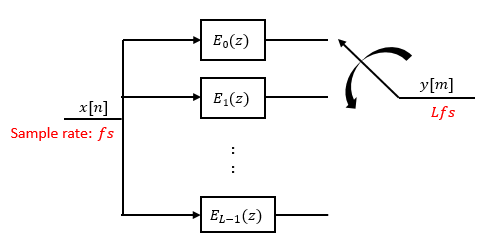# FIR Interpolation

Upsample and filter input signals

• Library:
• DSP System Toolbox / Filtering / Multirate Filters

DSP System Toolbox HDL Support / Filtering

•## Description

The FIR Interpolation block upsamples an input by the integer upsampling factor L along the first dimension. The FIR interpolator (as shown in the schematic) conceptually consists of an upsampler followed by an FIR anti-imaging filter, which is usually an approximation of an ideal band-limited interpolation filter. To design an FIR anti-imaging filter, use the `designMultirateFIR` function.

The upsampler upsamples each channel of the input to a higher rate by inserting L–1 zeros between samples. The FIR filter that follows filters each channel of the upsampled data. The resulting discrete-time signal has a sample rate that is L times the original sample rate.The actual block algorithm implements a direct-form FIR polyphase structure, an efficient equivalent of the combined system depicted in the diagram. For more details, see Algorithms.

You can use the FIR Interpolation block inside triggered subsystems when you set the Rate options parameter to `Enforce single-rate processing`.

Under specific conditions, this block also supports SIMD code generation. For more details, see Code Generation.

## Ports

### Input

expand all

Specify the data input as a vector or a matrix.

This block supports variable-size inputs. That is, the frame size (number of rows) of the signal can change during simulation but the number of channels cannot.

This port is unnamed until you set Coefficient source to Input port.

Data Types: `single` | `double` | `int8` | `int16` | `int32` | `uint8` | `uint16` | `uint32` | `fixed point`
Complex Number Support: Yes

Specify the numerator coefficients of the FIR filter as a vector.

The transfer function H(z) of the FIR filter is given by:

`$H\left(z\right)={b}_{0}+{b}_{1}{z}^{-1}+...+{b}_{N}{z}^{-N}$`

You can generate the FIR filter coefficient vector, b = [b0, b1, …, bN], using one of the DSP System Toolbox™ filter design functions such as `designMultirateFIR`, `firnyquist`, `firhalfband`, `firgr` or `firceqrip`.

To act as an effective anti-imaging filter, the coefficients usually correspond to a lowpass filter with a normalized cutoff frequency no greater than the reciprocal of the interpolation factor. To design such a filter, use the `designMultirateFIR` function.

Coefficient values are tunable. That is, their values can change during simulation while their properties such as size, data type, and complexity cannot change.

The data type of the Num input must match the data type of the In input.

#### Dependencies

The Num input port appears when you set Coefficient source as Input port.

Data Types: `single` | `double` | `int8` | `int16` | `int32` | `uint8` | `uint16` | `uint32` | `fixed point`
Complex Number Support: Yes

### Output

expand all

Output of the FIR Interpolator block, returned as a vector or a matrix.

When Rate options is set to:

• `Enforce single-rate processing` — When you select this option, the block maintains the input sample rate, and interpolates the signal by increasing the output frame size by a factor of L.

• `Allow multirate processing` — When you select this option, the block interpolates the signal such that the output sample rate is L times faster than the input sample rate.

This port is unnamed until you set Coefficient source to Input port.

Data Types: `single` | `double` | `int8` | `int16` | `int32` | `uint8` | `uint16` | `uint32` | `fixed point`
Complex Number Support: Yes

## Parameters

expand all

Coefficient source

Specify the FIR filter coefficient source as one of the following:

• Dialog parameters –– Specify the filter coefficients through the FIR filter coefficients parameter in the block dialog box.

• Input port –– Specify the filter coefficients through the Num input port.

• Filter object –– Specify the filter using a `dsp.FIRInterpolator` System object™.

• Auto –– When you select Auto, the block designs an FIR interpolator using the interpolation factor you specify in Interpolation factor. The `designMultirateFIR` function designs the filter and returns the coefficients used by the block.

Main Tab

Specify the numerator coefficients of the FIR filter transfer function H(z).

`$H\left(z\right)={b}_{0}+{b}_{1}{z}^{-1}+...+{b}_{N}{z}^{-N}$`

You can generate the FIR filter coefficient vector, b = [b0, b1, …, bN], using one of the DSP System Toolbox filter design functions such as `designMultirateFIR`, `firnyquist`, `firhalfband`, `firgr` or `firceqrip`.

To act as an effective anti-imaging filter, the coefficients usually correspond to a lowpass filter with a normalized cutoff frequency no greater than the reciprocal of the interpolation factor. To design such a filter, use the `designMultirateFIR` function.

The block internally initializes all filter states to zero.

#### Dependencies

This parameter appears only when you set the Coefficient source to Dialog parameters.

Data Types: `single` | `double` | `int8` | `int16` | `int32` | `int64` | `uint8` | `uint16` | `uint32` | `uint64`
Complex Number Support: Yes

Specify the integer factor L. The block increases the sample rate of the input sequence by this factor.

#### Dependencies

This parameter appears only when you set the Coefficient source to Dialog parameters, Input port, or Auto.

Data Types: `single` | `double` | `int8` | `int16` | `int32` | `int64` | `uint8` | `uint16` | `uint32` | `uint64`

Specify the name of the multirate filter object that you want the block to implement. You must specify the filter as a `dsp.FIRInterpolator` System object.

You can define the System object directly in the block dialog box. Alternatively, you can define the object in a MATLAB® workspace variable and specify the variable in the block dialog box.

For information on creating System objects, see Define Basic System Objects.

#### Dependencies

This parameter appears only when you set the Coefficient source to Filter object.

Specify how the block should process the input. You can set this parameter to one of the following options:

• `Columns as channels (frame based)` — When you select this option, the block treats each column of the input as a separate channel.

• `Elements as channels (sample based)` — When you select this option, the block treats each element of the input as a separate channel.

Specify the method by which the block should interpolate the input. You can select one of the following options:

• `Enforce single-rate processing` — When you select this option, the block maintains the input sample rate, and interpolates the signal by increasing the output frame size by a factor of L. To select this option, you must set the Input processing parameter to ```Columns as channels (frame based)```.

• `Allow multirate processing` — When you select this option, the block interpolates the signal such that the output sample rate is L times faster than the input sample rate.

When you set the Rate options parameter to ```Allow multirate processing``` and run your models in Simulink® `MultiTasking` mode, the block exhibits latency. The amount of latency for multirate, multitasking operation depends on how you set the Input processing parameter.

Input processingLatency

```Elements as channels (sample based)```

L samples

```Columns as channels (frame based)```

L frames (Ki samples per frame)

When the block exhibits latency, the default initial condition is zero. Alternatively, you can use the Output buffer initial conditions parameter to specify a matrix of initial conditions containing one value for each channel or a scalar initial condition that the block applies to all channels. The block divides the Output buffer initial conditions by the Interpolation factor and outputs the scaled initial conditions until the first filtered input sample becomes available.

Output buffer initial conditions are stored in the output data type and scaling.

#### Dependencies

This parameter appears only when you configure the block to perform multirate processing by setting Rate options to `Allow multirate processing`.

Data Types: `single` | `double` | `int8` | `int16` | `int32` | `int64` | `uint8` | `uint16` | `uint32` | `uint64`
Complex Number Support: Yes

Click on this button to open the Filter Visualization Tool (`fvtool`) and display the filter response of the filter defined in the block dialog box.

Data Types Tab

Select the rounding mode for fixed-point operations. The default is `Floor`. The filter coefficients do not obey this parameter and always round to `Nearest`.

Note

The Rounding mode and Saturate on integer overflow settings have no effect on numerical results when all the following conditions exist:

• Product output is ```Inherit: Inherit via internal rule```

• Accumulator is ```Inherit: Inherit via internal rule```

• Output is ```Inherit: Same as accumulator```

With these data type settings, the block is effectively operating in the full-precision mode.

When you select this parameter, the block saturates the result of its fixed-point operation. When you clear this parameter, the block wraps the result of its fixed-point operation. For details on `saturate` and `wrap`, see overflow mode for fixed-point operations.

Note

The Rounding mode and Saturate on integer overflow parameters have no effect on numeric results when all these conditions are met:

• Product output data type is ```Inherit: Inherit via internal rule```.

• Accumulator data type is ```Inherit: Inherit via internal rule```.

With these data type settings, the block operates in the full-precision mode.

Specify the coefficients data type. See Fixed Point and Multiplication Data Types for illustrations depicting the use of the coefficients data type in this block.

You can set this parameter to one of the following:

• ```Inherit: Same word length as input```

• `fixdt(1,16,0)` or `fixdt(1,16)` –– Specify a data type object.

Click the buttonto display the Data Type Assistant, which helps you set the Coefficients parameter.

#### Dependencies

This parameter appears only when you set Coefficient source to `Dialog parameters`, `Filter object`, or `Auto`.

When Coefficient source is set to `Filter object`, Coefficients parameter is automatically set to `Same word length as input`.

Specify the minimum value of the filter coefficients. The default value is `[]` (unspecified). Simulink software uses this value to perform automatic scaling of fixed-point data types.

#### Dependencies

This parameter appears only when you set Coefficient source to `Dialog parameters` or `Auto`.

Specify the maximum value of the filter coefficients. The default value is `[]` (unspecified). Simulink software uses this value to perform automatic scaling of fixed-point data types.

#### Dependencies

This parameter appears only when you set Coefficient source to `Dialog parameters` or `Auto`.

Specify the product output data type. See Fixed Point and Multiplication Data Types for illustrations depicting the use of the product output data type in this block.

You can set this parameter to one of the following:

• ```Inherit: Inherit via internal rule```

• `Inherit: Same as input`

• `fixdt(1,16,0)` –– Specify a data type object.

Click the buttonto display the Data Type Assistant, which helps you set the Product output parameter.

#### Dependencies

When Coefficient source is set to `Filter object`, Product output parameter is automatically set to ```Full precision```.

Specify the accumulator data type. See Fixed Point for illustrations depicting the use of the accumulator data type in this block.

You can set this parameter to one of the following:

• `Inherit: Inherit via internal rule`.

• `Inherit: Same as input`

• `Inherit: Same as product output`

• `fixdt(1,16,0)` –– Specify a data type object.

Click the buttonto display the Data Type Assistant, which helps you set the Accumulator parameter.

#### Dependencies

When Coefficient source is set to `Filter object`, Accumulator parameter is automatically set to `Full precision`.

Specify the output data type. See Fixed Point for illustrations depicting the use of the output data type in this block.

You can set it to one of the following:

• `Inherit: Same as accumulator`

• `Inherit: Same as input`

• `Inherit: Same as product output`

• `fixdt(1,16,0)` –– Specify a data type object.

Click the buttonto display the Data Type Assistant, which helps you set the Output parameter.

#### Dependencies

When Coefficient source is set to `Filter object`, Output parameter is automatically set to `Same as accumulator`.

Specify the minimum value that the block should output. The default value is `[]` (unspecified). Simulink software uses this value to perform:

• Simulation range checking (see Specify Signal Ranges (Simulink))

• Automatic scaling of fixed-point data types

#### Dependencies

This parameter appears only when you set Coefficient source to `Dialog parameters`, `Input port`, or `Auto`.

Specify the maximum value that the block should output. The default value is `[]` (unspecified). Simulink software uses this value to perform:

• Simulation range checking (see Specify Signal Ranges (Simulink))

• Automatic scaling of fixed-point data types

#### Dependencies

This parameter appears only when you set Coefficient source to `Dialog parameters`, `Input port`, or `Auto`.

Select this parameter to prevent the fixed-point tools from overriding the data types you specify in the block dialog box.

## Block Characteristics

 Data Types `double` | `fixed point` | `integer` | `single` Direct Feedthrough `no` Multidimensional Signals `no` Variable-Size Signals `yes` Zero-Crossing Detection `no`

expand all

## Algorithms

The FIR interpolation filter is implemented efficiently using a polyphase structure.

To derive the polyphase structure, start with the transfer function of the FIR filter:

`$H\left(z\right)={b}_{0}+{b}_{1}{z}^{-1}+...+{b}_{N}{z}^{-N}$`

N+1 is the length of the FIR filter.

You can rearrange this equation as follows:

`$H\left(z\right)=\begin{array}{c}\left({b}_{0}+{b}_{L}{z}^{-L}+{b}_{2L}{z}^{-2L}+..+{b}_{N-L+1}{z}^{-\left(N-L+1\right)}\right)+\\ {z}^{-1}\left({b}_{1}+{b}_{L+1}{z}^{-L}+{b}_{2L+1}{z}^{-2L}+..+{b}_{N-L+2}{z}^{-\left(N-L+1\right)}\right)+\\ \begin{array}{c}⋮\\ {z}^{-\left(L-1\right)}\left({b}_{L-1}+{b}_{2L-1}{z}^{-L}+{b}_{3L-1}{z}^{-2L}+..+{b}_{N}{z}^{-\left(N-L+1\right)}\right)\end{array}\end{array}$`

L is the number of polyphase components, and its value equals the interpolation factor that you specify.

You can write this equation as:

`$H\left(z\right)={E}_{0}\left({z}^{L}\right)+{z}^{-1}{E}_{1}\left({z}^{L}\right)+...+{z}^{-\left(L-1\right)}{E}_{L-1}\left({z}^{L}\right)$`

E0(zL), E1(zL), ..., EL-1(zL) are polyphase components of the FIR filter H(z).

Conceptually, the FIR interpolation filter contains an upsampler followed by an FIR lowpass filter H(z).Replace H(z) with its polyphase representation.Here is the multirate noble identity for interpolation.Applying the noble identity for interpolation moves the upsampling operation to after the filtering operation. This move enables you to filter the signal at a lower rate.You can replace the upsampling operator, delay block, and adder with a commutator switch. The switch starts on the first branch 0 and moves in the counterclockwise direction, each time receiving one sample from each branch. The interpolator effectively outputs L samples for every one input sample it receives. Hence the sample rate at the output of the FIR interpolation filter is Lfs.Fliege, N. J. Multirate Digital Signal Processing: Multirate Systems, Filter Banks, Wavelets . West Sussex, England: John Wiley & Sons, 1994.

 Orfanidis, Sophocles J. Introduction to Signal Processing . Upper Saddle River, NJ: Prentice-Hall, 1996.

## SupportGet trial now